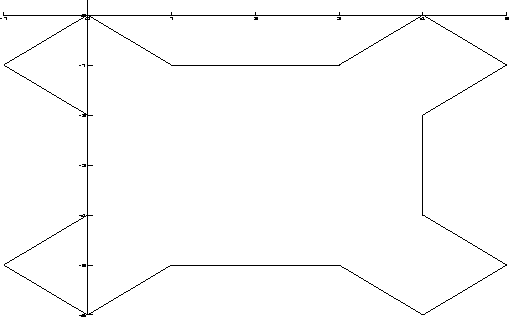Next: Plotting a Function and Up: Creating Plots Previous: Creating Plots

Plotting a Set of Points

There are two ways in which we can plot a set of points in Darwin. We can plot a set of magnitudes by using DrawPlot as follows:
DrawPlot(t : list(real))
Darwin connects consecutive points of t with a line. The x-axis is labeled with 1..length(t) and the y-axis is labeled with the elements of t.

> DrawPlot([0.01, 0.08, 0.21, 0.41, 0.19, 0.09, 0.02]);
We can now view and print the results by executing the commands
% ghostview temp.ps
% lpr temp.ps
directly from within the UNIX environment. Other operating systems will require different commands for viewing and printing your graphs. From within Darwin, we can view the contents of temp.ps by executing
> ViewPlot();
This command only displays the file named temp.ps located in the current directory.

If, instead, we would rather plot a set of points in the plane, we use DrawPlot as follows:
DrawPlot(t : list(real, real))
Each element t=[u,v] specifies a point in the Cartesian (xy-plane). Consecutive points are connected with a line.

> sierpinsky := [[0,0], [1,-1], [3,-1], [4,0], [5,-1], [4,-2],
> [4,-4], [5,-5], [4,-6], [3, -5], [1, -5], [0,-6], [-1,-5],
> [0,-4], [0,-2], [-1,-1], [0,0]]:
> DrawPlot(sierpinsky);
The plot of sierpinsky is shown in Figure.Next: Plotting a Function and Up: Creating Plots Previous: Creating Plots
Gaston Gonnet
1998-09-15